# Test: Probability- 2

## 10 Questions MCQ Test UPSC Prelims Paper 2 CSAT - Quant, Verbal & Decision Making | Test: Probability- 2

Description
Attempt Test: Probability- 2 | 10 questions in 10 minutes | Mock test for Banking Exams preparation | Free important questions MCQ to study UPSC Prelims Paper 2 CSAT - Quant, Verbal & Decision Making for Banking Exams Exam | Download free PDF with solutions
QUESTION: 1

### What is the probability of selecting a prime number from 1,2,3,... 10 ?

Solution:

Total count of numbers, n(S) = 10

Prime numbers in the given range are 2,3,5 and 7
Hence, total count of prime numbers in the given range, n(E) = 4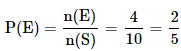QUESTION: 2

### 3 balls are drawn randomly from a bag contains 3 black, 5 red and 4 blue balls. What is the probability that the balls drawn contain balls of different colors?

Solution:

Total number of balls = 3 + 5 + 4 = 12

Let S be the sample space.
n(S) = Total number of ways of drawing 3 balls out of 12 = 12C3

Let E = Event of drawing 3 different coloured balls

To get 3 different coloured balls,we need to select one black ball from 3 black balls,
one red ball from 5 red balls, one blue ball from 4 blue balls

Number of ways in which this can be done = 3C1 × 5C1 × 4C1
i.e., n(E) = 3C1 × 5C1 × 4C1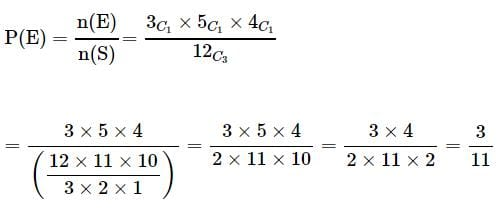QUESTION: 3

### 5 coins are tossed together. What is the probability of getting exactly 2 heads?

Solution:

Total number of outcomes possible when a coin is tossed = 2 (? Head or Tail)
Hence, total number of outcomes possible when 5 coins are tossed, n(S) = 25

E = Event of getting exactly 2 heads when 5 coins are tossed
n(E) = Number of ways of getting exactly 2 heads when 5 coins are tossed = 5C2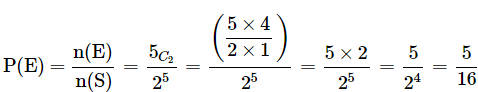QUESTION: 4

What is the probability of drawing a "Queen" from a deck of 52 cards?

Solution:

Total number of cards, n(S) = 52

Total number of "Queen" cards, n(E) = 4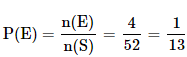QUESTION: 5

A card is randomly drawn from a deck of 52 cards. What is the probability getting an Ace or King or Queen?

Solution:

Total number of cards = 52

Total number of Ace cards = 4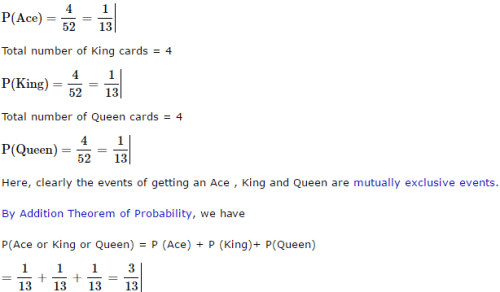QUESTION: 6

A card is randomly drawn from a deck of 52 cards. What is the probability getting a five of Spade or Club?

Solution: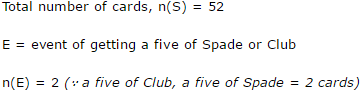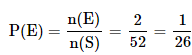QUESTION: 7

When two dice are rolled, what is the probability that the sum is either 7 or 11?

Solution:

Total number of outcomes possible when a die is rolled = 6 (? any one face out of the 6 faces)

Hence, total number of outcomes possible when two dice are rolled = 6 × 6 = 36

To get a sum of 7, the following are the favourable cases.
(1, 6), (2, 5), {3, 4}, (4, 3), (5, 2), (6,1)

=> Number of ways in which we get a sum of 7 = 6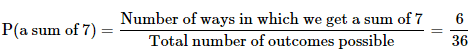To get a sum of 11, the following are the favourable cases. (5, 6), (6, 5) => Number of ways in which we get a sum of 11 = 2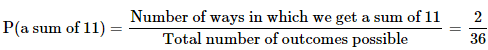Here, clearly the events are mutually exclusive events. By Addition Theorem of Probability, we have P(a sum of 7 or a sum of 11) = P(a sum of 7) + P( a sum of 11)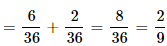QUESTION: 8

A card is randomly drawn from a deck of 52 cards. What is the probability getting either a King or a Diamond?

Solution:

Total number of cards = 52

Total Number of King Cards = 4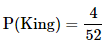Total Number of Diamond Cards = 13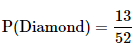Total Number of Cards which are both King and Diamond = 1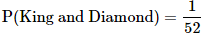Here a card can be both a Diamond card and a King. Hence these are not mutually exclusive events. (Reference : mutually exclusive events) . By Addition Theorem of Probability, we have P(King or a Diamond) = P(King) + P(Diamond) – P(King and Diamond)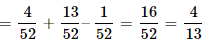QUESTION: 9

John and Dani go for an interview for two vacancies. The probability for the selection of John is 1/3 and whereas the probability for the selection of Dani is 1/5. What is the probability that none of them are selected?

Solution: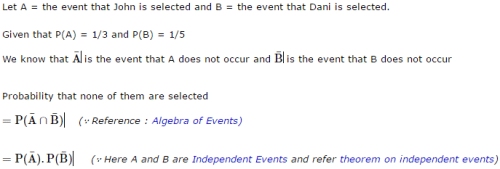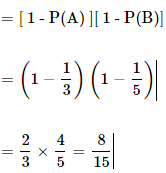QUESTION: 10

John and Dani go for an interview for two vacancies. The probability for the selection of John is 1/3 and whereas the probability for the selection of Dani is 1/5. What is the probability that only one of them is selected?

Solution:

The correct answer is B as
probability of one of them being selected, imply either John must be selected and Dani must not or Dani must be selected and John must not.
The probability of John's selection is 1/3, so that of his disselection is (1-1/3) = 2/3.
The probability of Dani's selection is 1/5, so that of his disselection is (1-1/5) = 4/5.
The probability of John's selection and Dani's disselection is 1/3 x 4/5 = 4/15.
The probability of Dani's selection and John's disselection is 2/3 x 1/5 = 2/15.
So the required probability is 4/15 + 2/15 = 6/15 = 2/5.Use Code STAYHOME200 and get INR 200 additional OFF Use Coupon Code